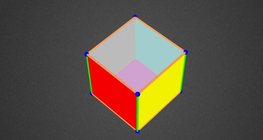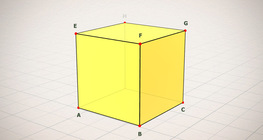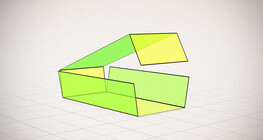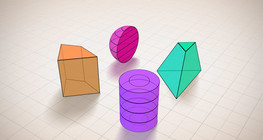Quantity: 0

Total: 0,00

0

# Notable products

### Notable products

A spectacular demonstration of algebraic expressions.

Mathematics

Keywords

notable products, (a-b)², (a+b)², (a+b)³, (a-b)³, a²-b², multiplication, formula, mathematics, algebra

### Related items#### Volume and surface area (exercise)

An exercise about the volume and surface area of solids generated from a ´base cube´.#### Coloring a cube

Coloring the vertices, edges and faces of a given cube according to the criteria specified in the exercise.#### Cube (exercises)

Edges, diagonals and faces of a cube can be identified by its vertices.#### Cube sections (exercise)

Examining solids formed by the intersection of a cube and a plane.#### Net of a cube (exercises)

Not all nets consisting of 6 congruent squares are foldable into cubes.#### Nets of a cuboid (exercises)

This animation demonstrates the different nets of a cuboid and includes a game.#### Shape games

Find views and nets of three-dimensional shapes.#### Views

Pick the shape that is shown in different views below.